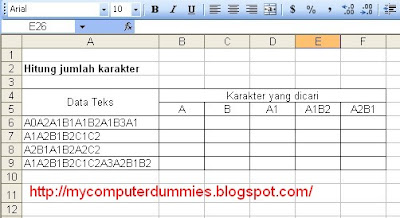## Tuesday, 28 July 2009

### Counting the number of characters

In the microsoft excel, you can count the number of characters (text or numbers), for example could see data table below
In column A is the set of characters, which will be selected and counted characters A, B, A1, A1B2, A2B1To calculate the character, then create the following fomula:
In cell B6, type the formula:
= LEN (A6) - LEN (Substitute (A6, "A ",""))
in C6 cell type the formula:
= LEN (A6) - LEN (Substitute (A6, "B ",""))
in D6 cell type the formula:
= (LEN (A6) - LEN (Substitute (A6, "A1 ","")))/ 2
in E6 cell type the formula:
= (LEN (A6) - LEN (Substitute (A6, "A1B2 ","")))/ 4
in F6 cell type the formula:
= (LEN (A6) - LEN (Substitute (A6, "A2B1 ","")))/ 4